GetFEM++  5.3
bgeot::polynomial< T > Class Template Reference

This class deals with plain polynomials with several variables. More...

#include <bgeot_poly.h>

Inherits vector< T >, and dal::static_stored_object.

## Public Member Functions

short_type degree () const
Gives the degree of the polynomial.

short_type real_degree () const
gives the degree of the polynomial, considering only non-zero coefficients

short_type dim () const
Gives the dimension (number of variables)

void change_degree (short_type dd)
Change the degree of the polynomial to d.

void add_monomial (const T &coeff, const power_index &power)
Add to the polynomial a monomial of coefficient a and correpsonding to the power index pi.

polynomialoperator+= (const polynomial &Q)
Add Q to P. P contains the result.

polynomialoperator-= (const polynomial &Q)
Subtract Q from P. P contains the result.

polynomial operator+ (const polynomial &Q) const
Add Q to P.

polynomial operator- (const polynomial &Q) const
Subtract Q from P.

polynomialoperator*= (const polynomial &Q)
Multiply P with Q. P contains the result.

polynomial operator* (const polynomial &Q) const
Multiply P with Q.

void direct_product (const polynomial &Q)
Product of P and Q considering that variables of Q come after variables of P. More...

polynomialoperator*= (const T &e)
Multiply P with the scalar a. P contains the result.

polynomial operator* (const T &e) const
Multiply P with the scalar a.

polynomialoperator/= (const T &e)
Divide P with the scalar a. P contains the result.

polynomial operator/ (const T &e) const
Divide P with the scalar a.

bool operator== (const polynomial &Q) const
operator ==.

bool operator!= (const polynomial &Q) const
operator !=.

void derivative (short_type k)
Derivative of P with respect to the variable k. P contains the result.

void one ()
Makes P = 1.

template<typename ITER >
eval (const ITER &it) const
Evaluate the polynomial. More...

polynomial ()
Constructor.

polynomial (short_type dim_, short_type degree_)
Constructor.

polynomial (short_type dim_, short_type degree_, short_type k)
Constructor for the polynomial 'x' (k=0), 'y' (k=1), 'z' (k=2) etc.

## Detailed Description

### template<typename T> class bgeot::polynomial< T >

This class deals with plain polynomials with several variables.

A polynomial of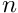variables and degree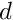is stored in a vector of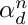components.

### Example of code

the following code is valid :

#include<bgeot_poly.h>
P = bgeot::polynomial<double>(2,2,1); // P = x
Q = bgeot::polynomial<double>(2,2,2); // Q = y
P += Q; // P is equal to x+y.
P *= Q; // P is equal to xy + y^2
bgeot::polynomial<double>::const_iterator ite = Q.end();
bgeot::polynomial<double>::const_iterator itb = Q.begin();
for ( ; itb != ite; ++itb, ++pi)
if (*itq != double(0))
cout "there is x to the power " << pi
<< " and y to the power "
<< pi << " and a coefficient " << *itq << endl;

### Monomials ordering.

 The constant coefficient is placed first with the index 0.
Two monomials of different degrees are ordered following
there respective degree.

If two monomials have the same degree, they are ordered with the
degree of the mononomials without the n firsts variables which
have the same degree. The index of the monomial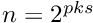is then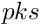where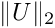is the degree of the monomial. (by convention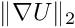).

### Dealing with the vector of power.

  The answer to the question : what is the next and previous
monomial of \form#30 in the
vector is the following :

To take the next coefficient, let \form#31 be the last index between 0
and \form#32 such that \form#33 ( \form#34 if there is not), then
make the operations \form#35@_fakenl.

To take the previous coefficient, let \form#31 be the last index
between 0 and \form#36 such that \form#33 (if there is not, there
is no previous monomial) then make the operations \form#37@_fakenl@_fakenl.


### Direct product multiplication.

  This direct product multiplication of P and Q is the
multiplication considering that the variables of Q follow the
variables of P. The result is a polynomial with the number of
variables of P plus the number of variables of Q.
The resulting polynomials have a smaller degree.

Definition at line 180 of file bgeot_poly.h.

## Member Function Documentation

template<typename T >
 void bgeot::polynomial< T >::direct_product ( const polynomial< T > & Q )

Product of P and Q considering that variables of Q come after variables of P.

P contains the result

Definition at line 383 of file bgeot_poly.h.

template<typename T >
template<typename ITER >
 T bgeot::polynomial< T >::eval ( const ITER & it ) const

Evaluate the polynomial.

"it" is an iterator pointing to the list of variables. A Horner scheme is used.

Definition at line 453 of file bgeot_poly.h.

The documentation for this class was generated from the following file: